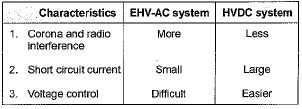Courses

# Test: HVDC of Power Systems

## 10 Questions MCQ Test Topicwise Question Bank for Electrical Engineering | Test: HVDC of Power Systems

Description
This mock test of Test: HVDC of Power Systems for Electrical Engineering (EE) helps you for every Electrical Engineering (EE) entrance exam. This contains 10 Multiple Choice Questions for Electrical Engineering (EE) Test: HVDC of Power Systems (mcq) to study with solutions a complete question bank. The solved questions answers in this Test: HVDC of Power Systems quiz give you a good mix of easy questions and tough questions. Electrical Engineering (EE) students definitely take this Test: HVDC of Power Systems exercise for a better result in the exam. You can find other Test: HVDC of Power Systems extra questions, long questions & short questions for Electrical Engineering (EE) on EduRev as well by searching above.
QUESTION: 1

Solution:
QUESTION: 2

### A dc line carries

Solution:

Due to requirement of less number of conductors, a dc line carries more power than a ac line, (losses of the line reduces in HVDC).

QUESTION: 3

### HVDC transmission is preferred to EHV AC because

Solution:

HVDC transmission is more reliable than EHV AC because it uses ground or sea return and therefore, in case of fault on a line it can still be used to supply power with the help of healthy lines.

QUESTION: 4

Back-to-back HVDC is used to

Solution:
QUESTION: 5

HVDC transmission needs

Solution:
QUESTION: 6

In HVDC system a converter acts as a rectifier when the firing angle α has a value

Solution:
QUESTION: 7

The capital cost on a HVDC converter is

Solution:
QUESTION: 8

In a dc transmision

Solution:

As operating frequency, f = 0 therefore, there is no effect of XL and XC.

QUESTION: 9

As compared to ac transmission dc transmission is free from

Solution:
QUESTION: 10

Which of the following is/are correctly matched?Solution: